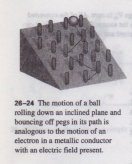# THEORY OF METALLIC CONDUCTION

THEORY OF METALLIC CONDUCTION

We can gain additional insight into electrical conduction by looking at the microscopic origin of conductivity. We’ll consider a very simple model that treats the electrons as classical particles and ignores their quantum-mechanical, wavelike behavior in solids. Using this model, we’ll derive an expression for the resistivity of a metal. Even though this model is not entirely correct conceptually, it will still help you to develop an intuitive idea of the microscopic basis of conduction. In the simplest microscopic model of conduction in a metal, each atom in the metallic crystal gives up one or more of its outer electrons. These electrons are then free to move through the crystal, colliding at intervals with the stationary positive ions.

The motion of the electrons is analogous to the motion of molecules of a gas moving through a porous bed of sand, and they are often referred to as an “electron gas.” If there is no electric field, the electrons move in straight lines between collisions,the directions of their velocities are random, and on average they never get anywhere  (Fig. 26-23a). But if an electric field is present, the paths curve slightly because of the acceleration caused by electric-field forces. Figure 26-23 b shows a few paths of an electron in an electric field directed from right to left. As we mentioned in Section 26-2, the average speed of random motion is of the order of 106 m Is, while the average drift speed is much smaller, of the order of 10-4m/s.

The average time between collisions is called the mean free time, denoted by T. Figure 26-24 (page 820) shows a mechanical analog of this electron motion. We would like to derive from this model an expression for the resistivity p of a material, defined by Eq. (26-5):where n is the number of free electrons per unit volume, q is the charge of  is their average drift velocity. (We also know that q = -e in an ordinary metal; that fact later.) We need to relate the drift velocity lid to the electric field E. The value of lid is determined by a steady-state condition in which, on average, the velocity gains of the charges due to the force of the E field are just balanced by the velocity losses due to collisions. To clarify this process, let’s imagine turning on the two effects one at a time. Suppose that before time t = 0 there is no field. The electron motion is then completely random.

A typical electron has velocity Vo at time t = 0, and the value averaged over many electrons (that is, the initial velocity of an average electron) is zero . Then at time t = 0 we turn on a constant electric field E. The field exerts a force F – qE on each charge, and this causes an acceleration ii in the direction of the force, given by If n and T are independent of E, then the resistivity is independent of E and the conducting material obeys Ohm’s law. Turning the interactions on one at a time may seem artificial. But a little thou shows that the derivation would come out the same if each electron had its own cI and the t = 0 times were different for different electrons. If T is the average time bet collisions, then  still the average electron drift velocity, even though the motions the various electrons aren’t actually correlated in the way we postulated.

What about the temperature dependence of resistivity? In a perfect crystal with atoms out of place, a correct quantum-mechanical analysis would let the free  move through the crystal with no collisions at all. But the atoms vibrate about their Librium positions. As the temperature increases, the amplitudes of these vib increase, collisions become more frequent, and the mean free time -r decreases. So this theory predicts that the resistivity of a metal increases with temperature. In a superconductor, roughly speaking, there are no inelastic collisions, -r is infinite, and the resistivity pis zero.